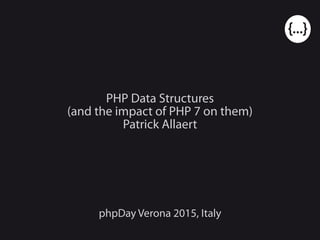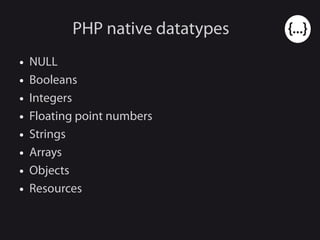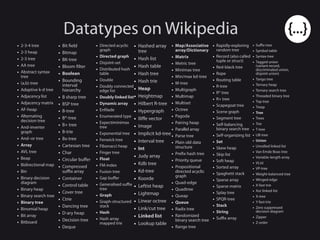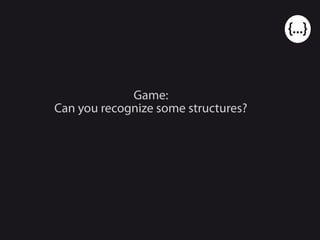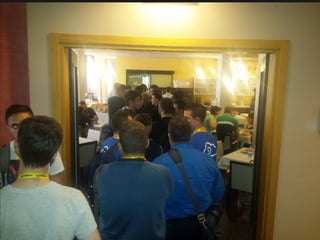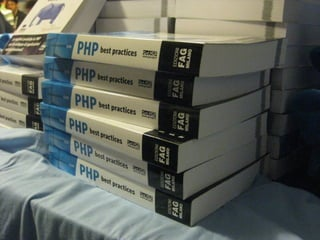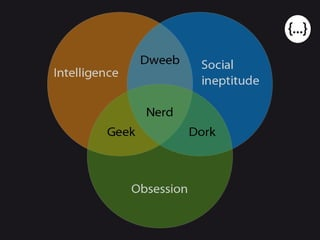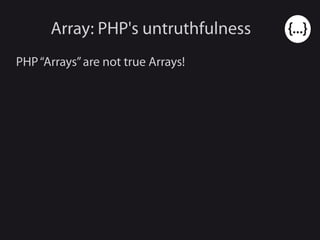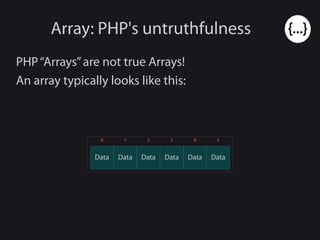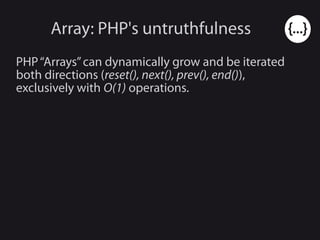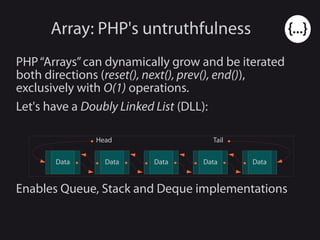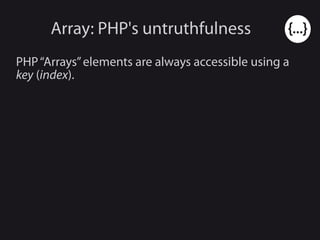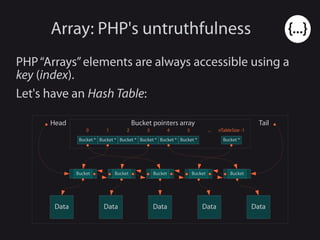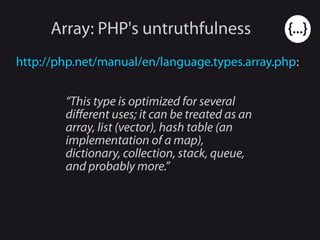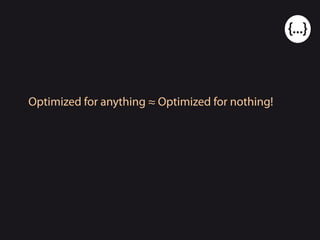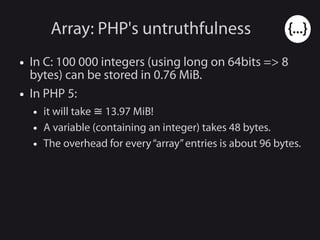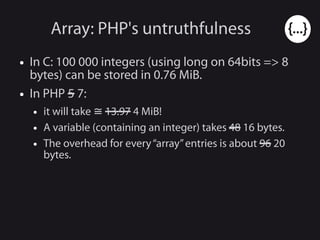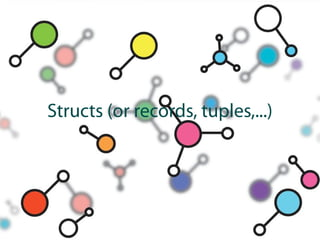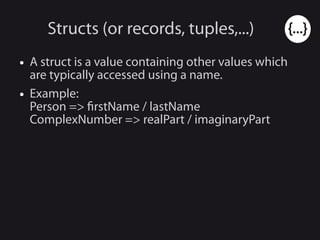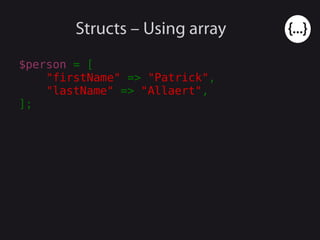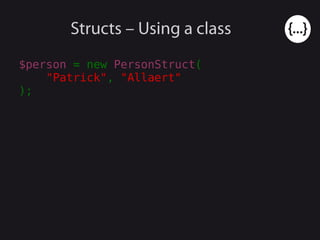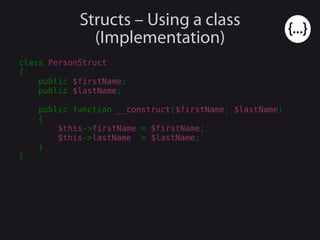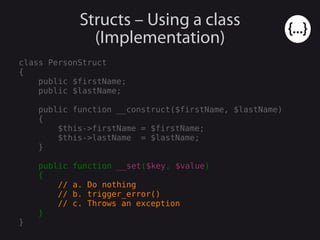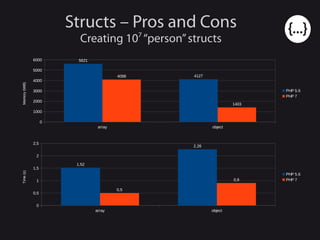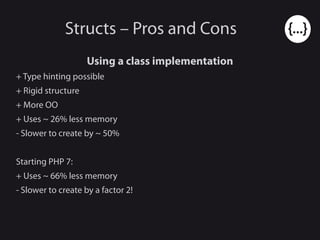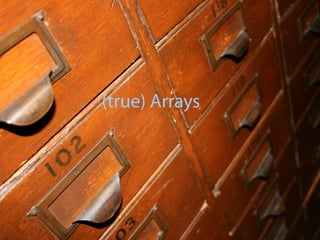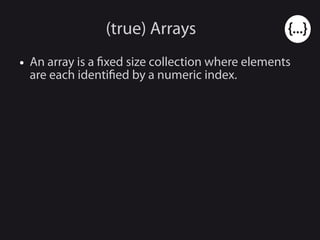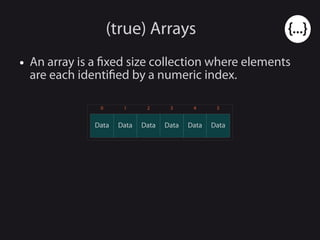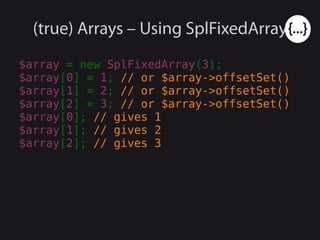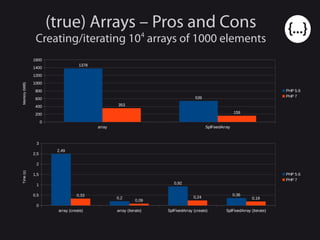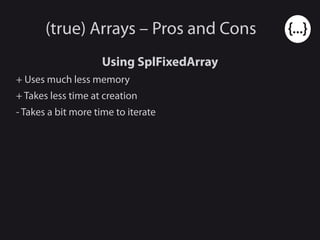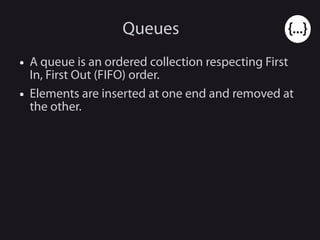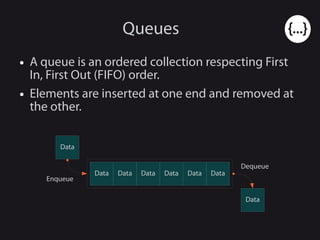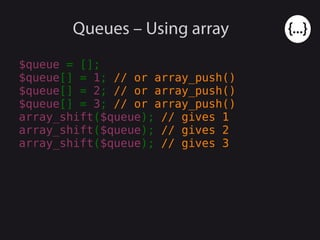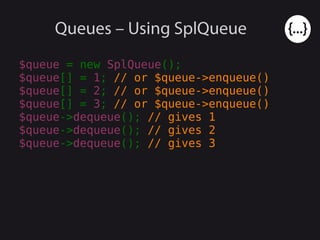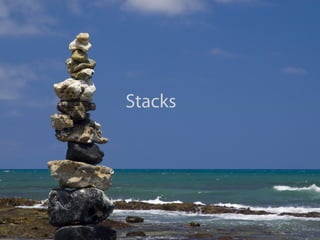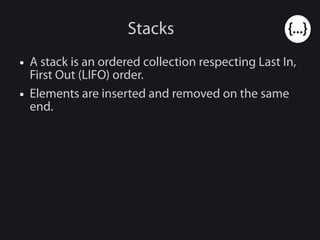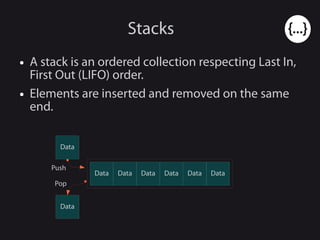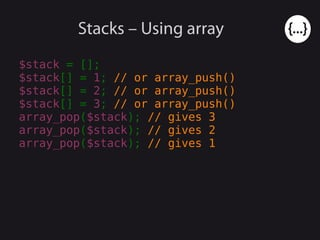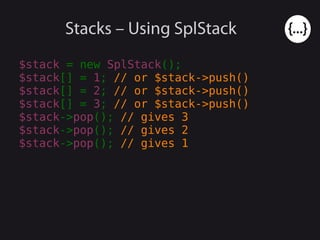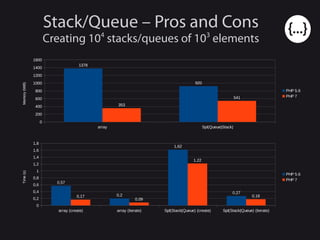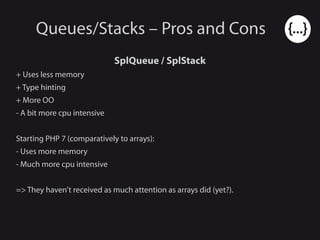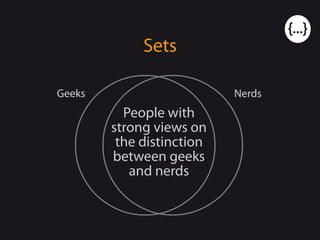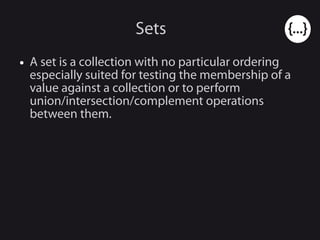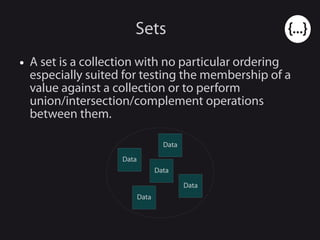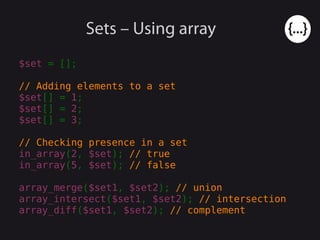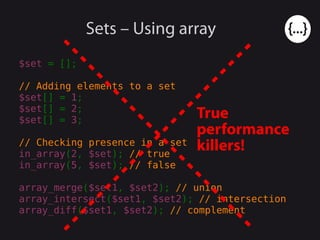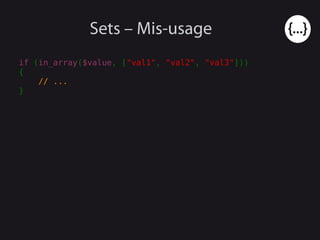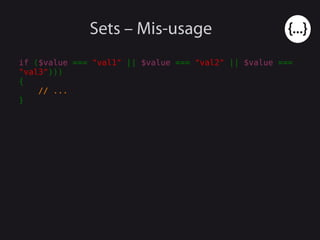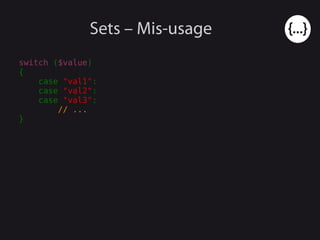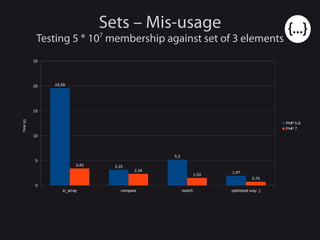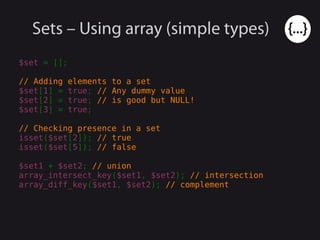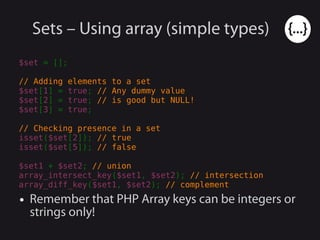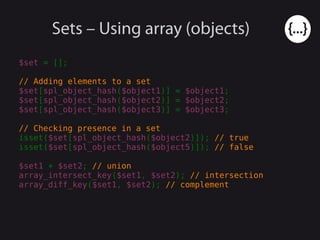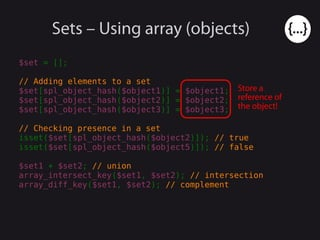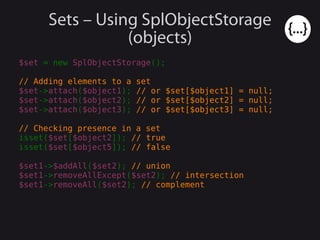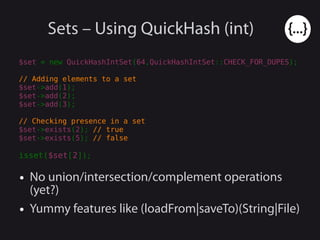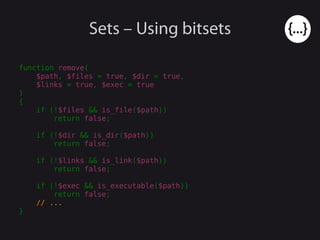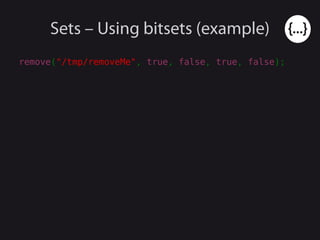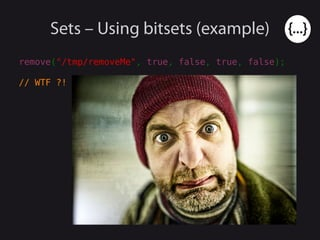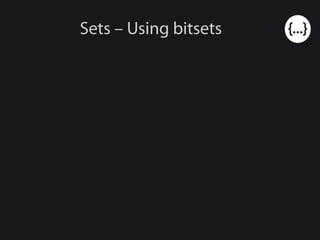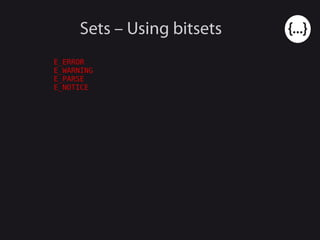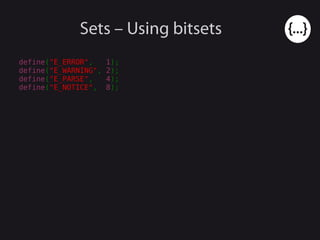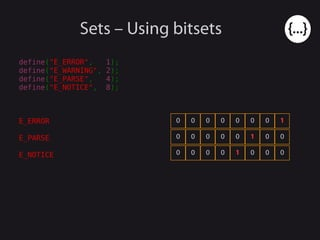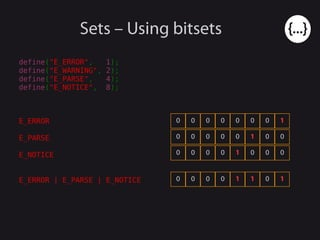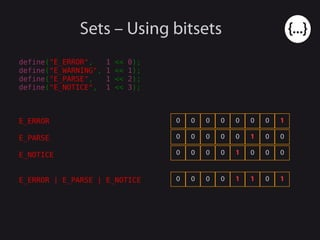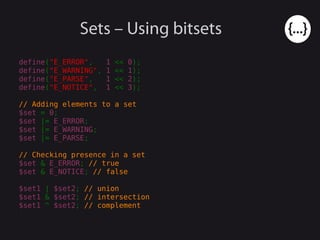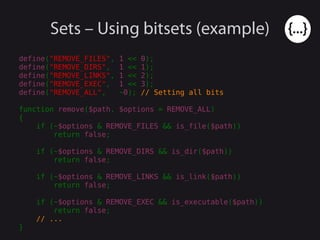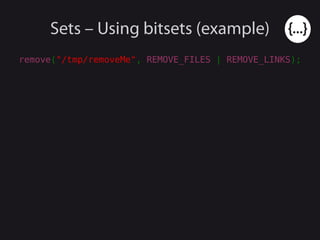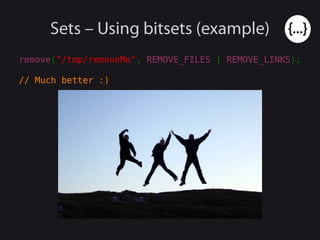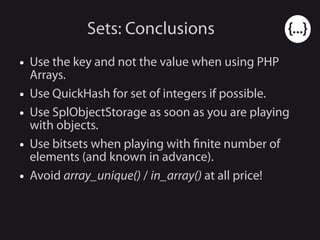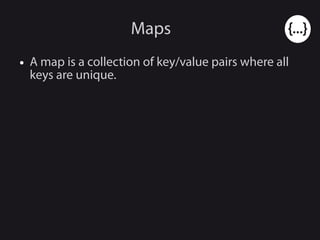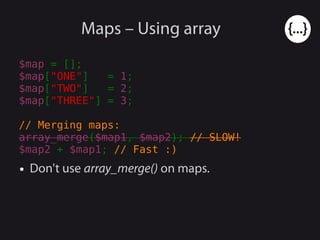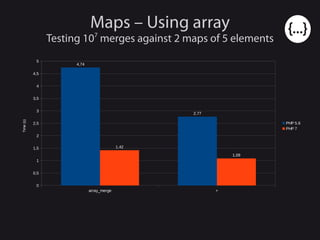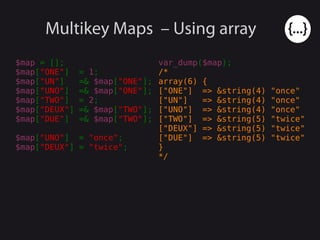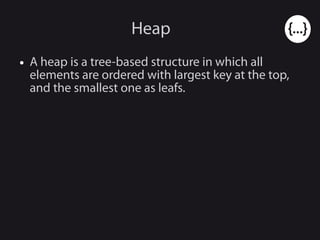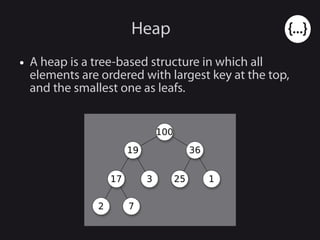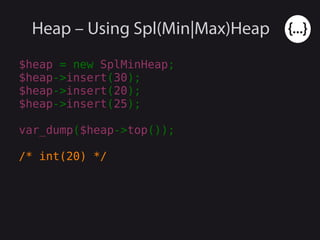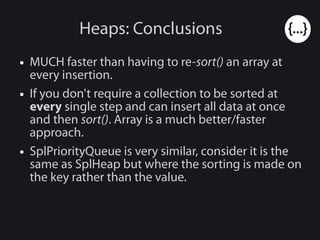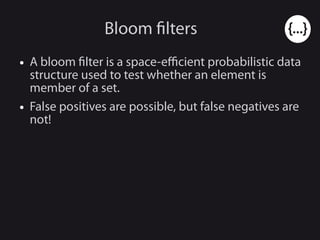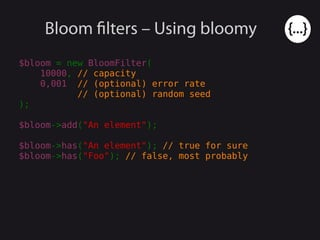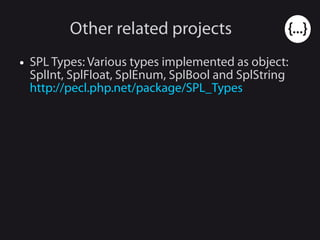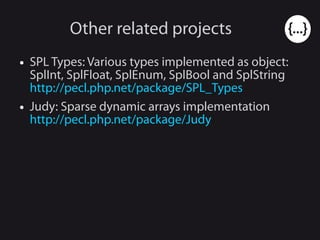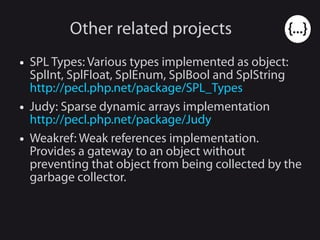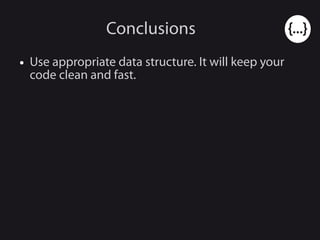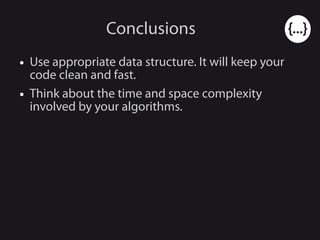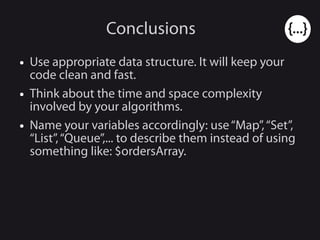1 of 95

### PHP data structures (and the impact of php 7 on them), phpDay Verona 2015, Italy

1. PHP Data Structures (and the impact of PHP 7 on them) Patrick Allaert phpDay Verona 2015, Italy
2. About me ● Patrick Allaert ● Founder of Libereco and co-founder of catchy.io ● Playing with PHP/Linux for +15 years ● eZ Publish core developer ● Author of the APM PHP extension ● @patrick_allaert ● patrickallaert@php.net ● http://github.com/patrickallaert/ ● http://patrickallaert.blogspot.com/
3. PHP native datatypes ● NULL ● Booleans ● Integers ● Floating point numbers ● Strings ● Arrays ● Objects ● Resources
5. Game: Can you recognize some structures?
6. Array: PHP's untruthfulness PHP“Arrays”are not true Arrays!
7. Array: PHP's untruthfulness PHP“Arrays”are not true Arrays! An array typically looks like this: Data DataDataData Data Data 0 1 2 3 4 5
8. Array: PHP's untruthfulness PHP“Arrays”can dynamically grow and be iterated both directions (reset(), next(), prev(), end()), exclusively with O(1) operations.
9. Array: PHP's untruthfulness PHP“Arrays”can dynamically grow and be iterated both directions (reset(), next(), prev(), end()), exclusively with O(1) operations. Let's have a Doubly Linked List (DLL): Data Data Data Data Data Head Tail Enables Queue, Stack and Deque implementations
10. Array: PHP's untruthfulness PHP“Arrays”elements are always accessible using a key (index).
11. Array: PHP's untruthfulness PHP“Arrays”elements are always accessible using a key (index). Let's have an Hash Table: Data Data Data Data Data Head Tail Bucket Bucket Bucket Bucket Bucket Bucket pointers array Bucket * 0 Bucket * 1 Bucket * 2 Bucket * 3 Bucket * 4 Bucket * 5 ... Bucket * nTableSize -1
12. Array: PHP's untruthfulness http://php.net/manual/en/language.types.array.php: “This type is optimized for several different uses; it can be treated as an array, list (vector), hash table (an implementation of a map), dictionary, collection, stack, queue, and probably more.”
13. Optimized for anything ≈ Optimized for nothing!
14. Optimized for anything ≈ Optimized for nothing!
15. Array: PHP's untruthfulness ● In C: 100 000 integers (using long on 64bits => 8 bytes) can be stored in 0.76 MiB. ● In PHP 5: ● it will take 13.97 MiB!≅ ● A variable (containing an integer) takes 48 bytes. ● The overhead for every“array”entries is about 96 bytes.
16. Array: PHP's untruthfulness ● In C: 100 000 integers (using long on 64bits => 8 bytes) can be stored in 0.76 MiB. ● In PHP 5 7: ● it will take ≅ 13.97 4 MiB! ● A variable (containing an integer) takes 48 16 bytes. ● The overhead for every“array”entries is about 96 20 bytes.
17. Data Structure
18. Structs (or records, tuples,...)
19. Structs (or records, tuples,...) ● A struct is a value containing other values which are typically accessed using a name. ● Example: Person => firstName / lastName ComplexNumber => realPart / imaginaryPart
20. Structs – Using array \$person = [ "firstName" => "Patrick", "lastName" => "Allaert", ];
21. Structs – Using a class \$person = new PersonStruct( "Patrick", "Allaert" );
22. Structs – Using a class (Implementation) class PersonStruct { public \$firstName; public \$lastName; public function __construct(\$firstName, \$lastName) { \$this->firstName = \$firstName; \$this->lastName = \$lastName; } }
23. Structs – Using a class (Implementation) class PersonStruct { public \$firstName; public \$lastName; public function __construct(\$firstName, \$lastName) { \$this->firstName = \$firstName; \$this->lastName = \$lastName; } public function __set(\$key, \$value) { // a. Do nothing // b. trigger_error() // c. Throws an exception } }
24. Structs – Pros and Cons Creating 107 “person”structs array object 0 1000 2000 3000 4000 5000 6000 5621 41274098 1403 PHP 5.6 PHP 7 Memory(MiB) array object 0 0,5 1 1,5 2 2,5 1,52 2,26 0,5 0,9 PHP 5.6 PHP 7 Time(s)
25. Structs – Pros and Cons Using a class implementation + Type hinting possible + Rigid structure + More OO + Uses ~ 26% less memory - Slower to create by ~ 50% Starting PHP 7: + Uses ~ 66% less memory - Slower to create by a factor 2!
26. (true) Arrays
27. (true) Arrays ● An array is a fixed size collection where elements are each identified by a numeric index.
28. (true) Arrays ● An array is a fixed size collection where elements are each identified by a numeric index. Data DataDataData Data Data 0 1 2 3 4 5
29. (true) Arrays – Using SplFixedArray \$array = new SplFixedArray(3); \$array = 1; // or \$array->offsetSet() \$array = 2; // or \$array->offsetSet() \$array = 3; // or \$array->offsetSet() \$array; // gives 1 \$array; // gives 2 \$array; // gives 3
30. (true) Arrays – Pros and Cons Creating/iterating 104 arrays of 1000 elements array SplFixedArray 0 200 400 600 800 1000 1200 1400 1600 1378 539 353 159 PHP 5.6 PHP 7 Memory(MiB) array (create) array (iterate) SplFixedArray (create) SplFixedArray (iterate) 0 0,5 1 1,5 2 2,5 3 2,49 0,2 0,92 0,360,33 0,09 0,24 0,19 PHP 5.6 PHP 7 Time(s)
31. (true) Arrays – Pros and Cons Using SplFixedArray + Uses much less memory + Takes less time at creation - Takes a bit more time to iterate
32. Queues
33. Queues ● A queue is an ordered collection respecting First In, First Out (FIFO) order. ● Elements are inserted at one end and removed at the other.
34. Queues ● A queue is an ordered collection respecting First In, First Out (FIFO) order. ● Elements are inserted at one end and removed at the other. Data DataDataData Data Data Data Data Enqueue Dequeue
35. Queues – Using array \$queue = []; \$queue[] = 1; // or array_push() \$queue[] = 2; // or array_push() \$queue[] = 3; // or array_push() array_shift(\$queue); // gives 1 array_shift(\$queue); // gives 2 array_shift(\$queue); // gives 3
36. Queues – Using SplQueue \$queue = new SplQueue(); \$queue[] = 1; // or \$queue->enqueue() \$queue[] = 2; // or \$queue->enqueue() \$queue[] = 3; // or \$queue->enqueue() \$queue->dequeue(); // gives 1 \$queue->dequeue(); // gives 2 \$queue->dequeue(); // gives 3
37. Stacks
38. Stacks ● A stack is an ordered collection respecting Last In, First Out (LIFO) order. ● Elements are inserted and removed on the same end.
39. Stacks ● A stack is an ordered collection respecting Last In, First Out (LIFO) order. ● Elements are inserted and removed on the same end. Data DataDataData Data Data Data Data Push Pop
40. Stacks – Using array \$stack = []; \$stack[] = 1; // or array_push() \$stack[] = 2; // or array_push() \$stack[] = 3; // or array_push() array_pop(\$stack); // gives 3 array_pop(\$stack); // gives 2 array_pop(\$stack); // gives 1
41. Stacks – Using SplStack \$stack = new SplStack(); \$stack[] = 1; // or \$stack->push() \$stack[] = 2; // or \$stack->push() \$stack[] = 3; // or \$stack->push() \$stack->pop(); // gives 3 \$stack->pop(); // gives 2 \$stack->pop(); // gives 1
42. Stack/Queue – Pros and Cons Creating 104 stacks/queues of 103 elements array Spl(Queue|Stack) 0 200 400 600 800 1000 1200 1400 1600 1378 920 353 541 PHP 5.6 PHP 7 Memory(MiB) array (create) array (iterate) Spl(Stack|Queue) (create) Spl(Stack|Queue) (iterate) 0 0,2 0,4 0,6 0,8 1 1,2 1,4 1,6 1,8 0,57 0,2 1,62 0,27 0,17 0,09 1,22 0,18 PHP 5.6 PHP 7 Time(s)
43. Queues/Stacks – Pros and Cons SplQueue / SplStack + Uses less memory + Type hinting + More OO - A bit more cpu intensive Starting PHP 7 (comparatively to arrays): - Uses more memory - Much more cpu intensive => They haven't received as much attention as arrays did (yet?).
44. Sets People with strong views on the distinction between geeks and nerds Geeks Nerds
45. Sets ● A set is a collection with no particular ordering especially suited for testing the membership of a value against a collection or to perform union/intersection/complement operations between them.
46. Sets ● A set is a collection with no particular ordering especially suited for testing the membership of a value against a collection or to perform union/intersection/complement operations between them. Data Data Data Data Data
47. Sets – Using array \$set = []; // Adding elements to a set \$set[] = 1; \$set[] = 2; \$set[] = 3; // Checking presence in a set in_array(2, \$set); // true in_array(5, \$set); // false array_merge(\$set1, \$set2); // union array_intersect(\$set1, \$set2); // intersection array_diff(\$set1, \$set2); // complement
48. Sets – Using array \$set = []; // Adding elements to a set \$set[] = 1; \$set[] = 2; \$set[] = 3; // Checking presence in a set in_array(2, \$set); // true in_array(5, \$set); // false array_merge(\$set1, \$set2); // union array_intersect(\$set1, \$set2); // intersection array_diff(\$set1, \$set2); // complement True performance killers!
49. Sets – Mis-usage if (in_array(\$value, ["val1", "val2", "val3"])) { // ... }
50. Sets – Mis-usage if (\$value === "val1" || \$value === "val2" || \$value === "val3"))) { // ... }
51. Sets – Mis-usage switch (\$value) { case "val1": case "val2": case "val3": // ... }
52. Sets – Mis-usage Testing 5 * 107 membership against set of 3 elements in_array compare switch optimized way ;) 0 5 10 15 20 25 19,59 3,15 5,2 1,97 3,43 2,34 1,53 0,75 PHP 5.6 PHP 7 Time(s)
53. Sets – Using array (simple types) \$set = []; // Adding elements to a set \$set = true; // Any dummy value \$set = true; // is good but NULL! \$set = true; // Checking presence in a set isset(\$set); // true isset(\$set); // false \$set1 + \$set2; // union array_intersect_key(\$set1, \$set2); // intersection array_diff_key(\$set1, \$set2); // complement
54. Sets – Using array (simple types) \$set = []; // Adding elements to a set \$set = true; // Any dummy value \$set = true; // is good but NULL! \$set = true; // Checking presence in a set isset(\$set); // true isset(\$set); // false \$set1 + \$set2; // union array_intersect_key(\$set1, \$set2); // intersection array_diff_key(\$set1, \$set2); // complement ● Remember that PHP Array keys can be integers or strings only!
55. Sets – Using array (objects) \$set = []; // Adding elements to a set \$set[spl_object_hash(\$object1)] = \$object1; \$set[spl_object_hash(\$object2)] = \$object2; \$set[spl_object_hash(\$object3)] = \$object3; // Checking presence in a set isset(\$set[spl_object_hash(\$object2)]); // true isset(\$set[spl_object_hash(\$object5)]); // false \$set1 + \$set2; // union array_intersect_key(\$set1, \$set2); // intersection array_diff_key(\$set1, \$set2); // complement
56. Sets – Using array (objects) \$set = []; // Adding elements to a set \$set[spl_object_hash(\$object1)] = \$object1; \$set[spl_object_hash(\$object2)] = \$object2; \$set[spl_object_hash(\$object3)] = \$object3; // Checking presence in a set isset(\$set[spl_object_hash(\$object2)]); // true isset(\$set[spl_object_hash(\$object5)]); // false \$set1 + \$set2; // union array_intersect_key(\$set1, \$set2); // intersection array_diff_key(\$set1, \$set2); // complement Store a reference of the object!
57. Sets – Using SplObjectStorage (objects) \$set = new SplObjectStorage(); // Adding elements to a set \$set->attach(\$object1); // or \$set[\$object1] = null; \$set->attach(\$object2); // or \$set[\$object2] = null; \$set->attach(\$object3); // or \$set[\$object3] = null; // Checking presence in a set isset(\$set[\$object2]); // true isset(\$set[\$object5]); // false \$set1->\$addAll(\$set2); // union \$set1->removeAllExcept(\$set2); // intersection \$set1->removeAll(\$set2); // complement
58. Sets – Using QuickHash (int) ● No union/intersection/complement operations (yet?) ● Yummy features like (loadFrom|saveTo)(String|File) \$set = new QuickHashIntSet(64,QuickHashIntSet::CHECK_FOR_DUPES); // Adding elements to a set \$set->add(1); \$set->add(2); \$set->add(3); // Checking presence in a set \$set->exists(2); // true \$set->exists(5); // false isset(\$set);
59. Sets – Using bitsets function remove( \$path, \$files = true, \$dir = true, \$links = true, \$exec = true ) { if (!\$files && is_file(\$path)) return false; if (!\$dir && is_dir(\$path)) return false; if (!\$links && is_link(\$path)) return false; if (!\$exec && is_executable(\$path)) return false; // ... }
60. Sets – Using bitsets (example) remove("/tmp/removeMe", true, false, true, false);
61. Sets – Using bitsets (example) remove("/tmp/removeMe", true, false, true, false); // WTF ?!
62. Sets – Using bitsets
63. Sets – Using bitsets E_ERROR E_WARNING E_PARSE E_NOTICE
64. Sets – Using bitsets define("E_ERROR", 1); define("E_WARNING", 2); define("E_PARSE", 4); define("E_NOTICE", 8);
65. Sets – Using bitsets define("E_ERROR", 1); define("E_WARNING", 2); define("E_PARSE", 4); define("E_NOTICE", 8); E_ERROR E_PARSE E_NOTICE 10000000 00100000 00010000
66. Sets – Using bitsets define("E_ERROR", 1); define("E_WARNING", 2); define("E_PARSE", 4); define("E_NOTICE", 8); E_ERROR E_PARSE E_NOTICE E_ERROR | E_PARSE | E_NOTICE 10000000 00100000 00010000 10110000
67. Sets – Using bitsets define("E_ERROR", 1 << 0); define("E_WARNING", 1 << 1); define("E_PARSE", 1 << 2); define("E_NOTICE", 1 << 3); E_ERROR E_PARSE E_NOTICE E_ERROR | E_PARSE | E_NOTICE 10000000 00100000 00010000 10110000
68. Sets – Using bitsets define("E_ERROR", 1 << 0); define("E_WARNING", 1 << 1); define("E_PARSE", 1 << 2); define("E_NOTICE", 1 << 3); // Adding elements to a set \$set = 0; \$set |= E_ERROR; \$set |= E_WARNING; \$set |= E_PARSE; // Checking presence in a set \$set & E_ERROR; // true \$set & E_NOTICE; // false \$set1 | \$set2; // union \$set1 & \$set2; // intersection \$set1 ^ \$set2; // complement
69. Sets – Using bitsets (example) define("REMOVE_FILES", 1 << 0); define("REMOVE_DIRS", 1 << 1); define("REMOVE_LINKS", 1 << 2); define("REMOVE_EXEC", 1 << 3); define("REMOVE_ALL", ~0); // Setting all bits function remove(\$path, \$options = REMOVE_ALL) { if (~\$options & REMOVE_FILES && is_file(\$path)) return false; if (~\$options & REMOVE_DIRS && is_dir(\$path)) return false; if (~\$options & REMOVE_LINKS && is_link(\$path)) return false; if (~\$options & REMOVE_EXEC && is_executable(\$path)) return false; // ... }
70. Sets – Using bitsets (example) remove("/tmp/removeMe", REMOVE_FILES | REMOVE_LINKS);
71. Sets – Using bitsets (example) remove("/tmp/removeMe", REMOVE_FILES | REMOVE_LINKS); // Much better :)
72. Sets: Conclusions ● Use the key and not the value when using PHP Arrays. ● Use QuickHash for set of integers if possible. ● Use SplObjectStorage as soon as you are playing with objects. ● Use bitsets when playing with finite number of elements (and known in advance). ● Avoid array_unique() / in_array() at all price!
73. Maps ● A map is a collection of key/value pairs where all keys are unique.
74. Maps – Using array ● Don't use array_merge() on maps. \$map = []; \$map["ONE"] = 1; \$map["TWO"] = 2; \$map["THREE"] = 3; // Merging maps: array_merge(\$map1, \$map2); // SLOW! \$map2 + \$map1; // Fast :)
75. Maps – Using array Testing 107 merges against 2 maps of 5 elements array_merge + 0 0,5 1 1,5 2 2,5 3 3,5 4 4,5 5 4,74 2,77 1,42 1,09 PHP 5.6 PHP 7 Time(s)
76. Multikey Maps – Using array \$map = []; \$map["ONE"] = 1; \$map["UN"] =& \$map["ONE"]; \$map["UNO"] =& \$map["ONE"]; \$map["TWO"] = 2; \$map["DEUX"] =& \$map["TWO"]; \$map["DUE"] =& \$map["TWO"]; \$map["UNO"] = "once"; \$map["DEUX"] = "twice"; var_dump(\$map); /* array(6) { ["ONE"] => &string(4) "once" ["UN"] => &string(4) "once" ["UNO"] => &string(4) "once" ["TWO"] => &string(5) "twice" ["DEUX"] => &string(5) "twice" ["DUE"] => &string(5) "twice" } */
77. Heap ● A heap is a tree-based structure in which all elements are ordered with largest key at the top, and the smallest one as leafs.
78. Heap ● A heap is a tree-based structure in which all elements are ordered with largest key at the top, and the smallest one as leafs.
79. Heap – Using Spl(Min|Max)Heap \$heap = new SplMinHeap; \$heap->insert(30); \$heap->insert(20); \$heap->insert(25); var_dump(\$heap->top()); /* int(20) */
80. Heaps: Conclusions ● MUCH faster than having to re-sort() an array at every insertion. ● If you don't require a collection to be sorted at every single step and can insert all data at once and then sort(). Array is a much better/faster approach. ● SplPriorityQueue is very similar, consider it is the same as SplHeap but where the sorting is made on the key rather than the value.
81. Bloom filters ● A bloom filter is a space-efficient probabilistic data structure used to test whether an element is member of a set. ● False positives are possible, but false negatives are not!
82. Bloom filters – Using bloomy \$bloom = new BloomFilter( 10000, // capacity 0,001 // (optional) error rate // (optional) random seed ); \$bloom->add("An element"); \$bloom->has("An element"); // true for sure \$bloom->has("Foo"); // false, most probably
83. Other related projects ● SPL Types: Various types implemented as object: SplInt, SplFloat, SplEnum, SplBool and SplString http://pecl.php.net/package/SPL_Types
84. Other related projects ● SPL Types: Various types implemented as object: SplInt, SplFloat, SplEnum, SplBool and SplString http://pecl.php.net/package/SPL_Types ● Judy: Sparse dynamic arrays implementation http://pecl.php.net/package/Judy
85. Other related projects ● SPL Types: Various types implemented as object: SplInt, SplFloat, SplEnum, SplBool and SplString http://pecl.php.net/package/SPL_Types ● Judy: Sparse dynamic arrays implementation http://pecl.php.net/package/Judy ● Weakref: Weak references implementation. Provides a gateway to an object without preventing that object from being collected by the garbage collector.
86. Conclusions ● Use appropriate data structure. It will keep your code clean and fast.
87. Conclusions ● Use appropriate data structure. It will keep your code clean and fast. ● Think about the time and space complexity involved by your algorithms.
88. Conclusions ● Use appropriate data structure. It will keep your code clean and fast. ● Think about the time and space complexity involved by your algorithms. ● Name your variables accordingly: use“Map”,“Set”, “List”,“Queue”,... to describe them instead of using something like: \$ordersArray.
89. Questions?
90. Thanks Don't forget to rate this talk on https://joind.in/14535 Stay in touch! @patrick_allaert patrickallaert@php.net
91. Photo Credits ● Tuned car: http://www.flickr.com/photos/gioxxswall/5783867752 ● London Eye Structure: http://www.flickr.com/photos/photographygal123/4883546484 ● Heap structure: http://en.wikipedia.org/wiki/File:Max-Heap.svg ● Drawers: http://www.flickr.com/photos/jamesclay/2312912612 ● Stones stack: http://www.flickr.com/photos/silent_e/2282729987 ● Tree: http://www.flickr.com/photos/drewbandy/6002204996